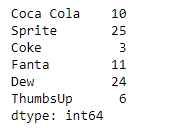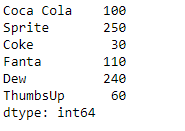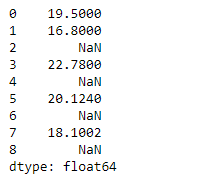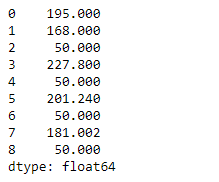GFG App
Open AppBrowser
Continue

# Python | Pandas Series.multiply()

Pandas series is a One-dimensional ndarray with axis labels. The labels need not be unique but must be a hashable type. The object supports both integer- and label-based indexing and provides a host of methods for performing operations involving the index.

Pandas` Series.multiply()` function perform the multiplication of series and other, element-wise. The operation is equivalent to `series * other`, but with support to substitute a fill_value for missing data in one of the inputs.

Syntax: Series.multiply(other, level=None, fill_value=None, axis=0)

Parameter :
other : Series or scalar value
fill_value : Fill existing missing (NaN) value
level : Broadcast across a level,

Returns : result : Series

Example #1: Use `Series.multiply()` function to perform the multiplication of a scalar with the given series object.

 `# importing pandas as pd ` `import` `pandas as pd ` ` `  `# Creating the Series ` `sr ``=` `pd.Series([``10``, ``25``, ``3``, ``11``, ``24``, ``6``]) ` ` `  `# Create the Index ` `index_ ``=` `[``'Coca Cola'``, ``'Sprite'``, ``'Coke'``, ``'Fanta'``, ``'Dew'``, ``'ThumbsUp'``] ` ` `  `# set the index ` `sr.index ``=` `index_ ` ` `  `# Print the series ` `print``(sr) `

Output :Now we will use `Series.multiply()` function to perform the multiplication of scalar with the series.

 `# multiply the given value with series ` `result ``=` `sr.multiply(other ``=` `10``) ` ` `  `# Print the result ` `print``(result) `

Output :As we can see in the output, the `Series.multiply()` function has returned the result of multiplication of the given scalar with the series object.

Example #2: Use `Series.multiply()` function to perform the multiplication of a scalar with the given series object. The given series object contains some missing values.

 `# importing pandas as pd ` `import` `pandas as pd ` ` `  `# Creating the Series ` `sr ``=` `pd.Series([``19.5``, ``16.8``, ``None``, ``22.78``, ``None``, ``20.124``, ``None``, ``18.1002``, ``None``]) ` ` `  `# Print the series ` `print``(sr) `

Output :Now we will use `Series.multiply()` function to perform the multiplication of scalar with the series.

 `# multiply the given value with series ` `# fill 5 at the place of all the missing values ` `result ``=` `sr.multiply(other ``=` `10``, fill_value ``=` `5``) ` ` `  `# Print the result ` `print``(result) `

Output :As we can see in the output, the `Series.multiply()` function has returned the result of multiplication of the given scalar with the series object.

My Personal Notes arrow_drop_up